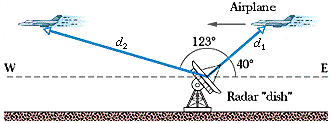Feb
11

# HW 2.2 Item #4

A radar station detects an airplane approaching directly from the east. At first observation, the range to the plane is d1 =390 m at 40° above the horizon. The airplane is tracked for another 123° in the vertical eastwest plane, the range at final contact being d2 = 770 m. See Fig. 4-30. Find the displacement of the airplane during the period of observation.My first thoughts on the problem were “Oh, its just the side of a triangle defined by d1 and d2 as the other two sides.  I can probably just use trig to figure it out geometrically.”  Well, this is true, but in order to work it out as a simple triangle, we would need the key piece of information that the plane is flying horizontally, with no change in elevation.  Unfortunately this is NOT true, as a closer look at the values will tell us.  We will get to that in a moment.  What we actually need to do is define displacement faithfully as the change in position: final position – initial position.

First, let’s analyze the positions determined by the radar:

Make a right triangle out of each vector and find the horizontal and vertical components.

d1x: 390 cos(40) = 299 m
d1y: 390 sin(40) = 251 m

d2x: 770 cos(17) = 736 m
d2y: 770 sin(17) = 225 m

Now find the change in the horizontal and vertical positions independently of each other:

Deltax = -736 – 299 = -1035 m
Deltay = 225-299 = -74 m  (clearly not the same height, so not flying horizontally as mentioned above)

Magnitude of displacement = sqrt (1035^2 + 74^2) = 1038 m
Direction of displacement = arctan (74/1035) = 4 degrees S of W, or 184 degrees

Now, there does seem to be a problem with the webassign answer on part b for some of your assignments, so I will throw out that part.  It does not seem to be coded correctly, but I can’t locate the error.  Sorry for any confusion.